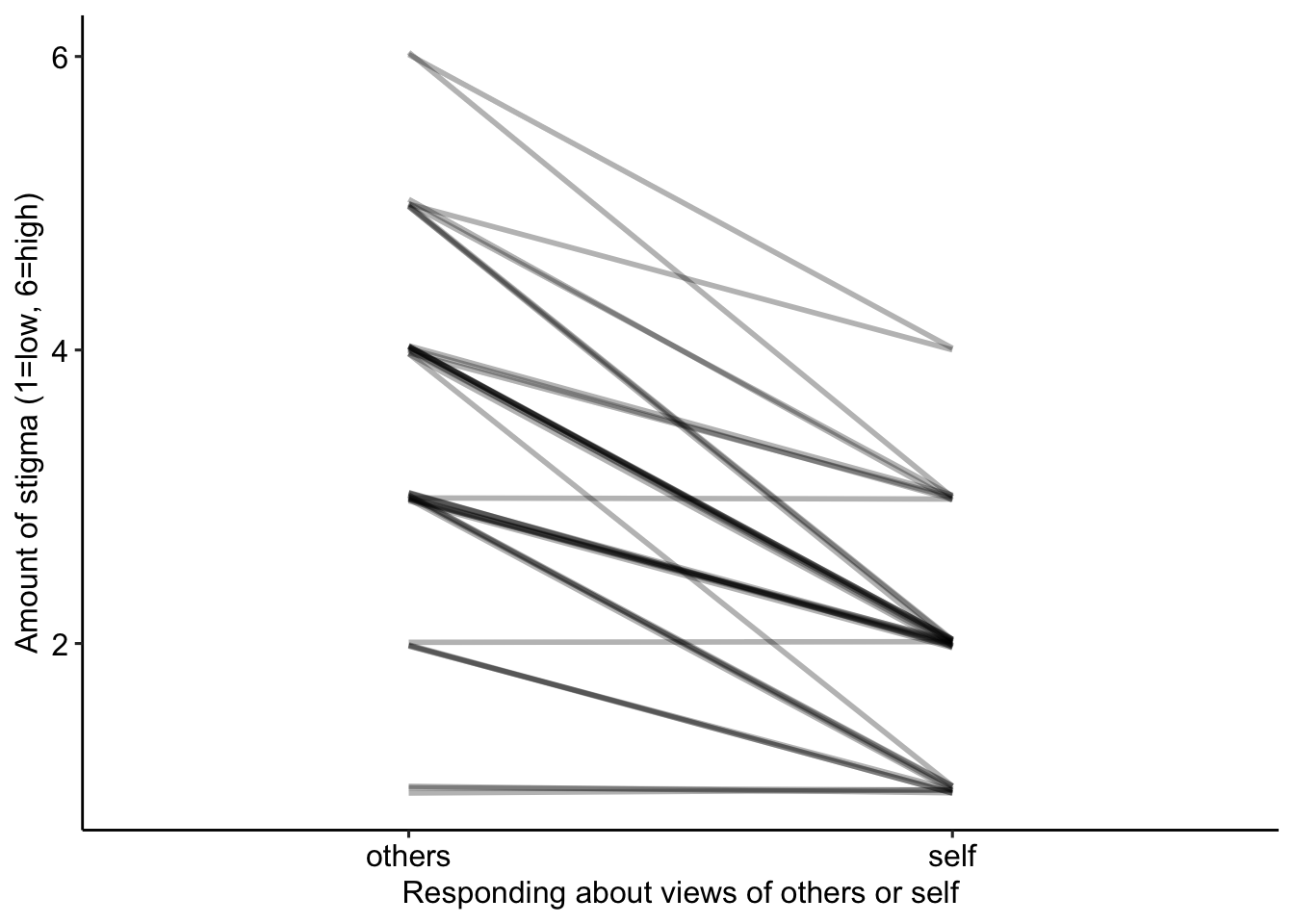ggplot scripts to draw figures like those in the Dynamic Ecology post Paired line plots (a.k.a. “reaction norms”) to visualize Likert data

library(ggplot2)
library(ggpubr)
library(data.table)

# make some fake data

set.seed(3)
n <- 40
self <- rbinom(n, 5, 0.25) + 1
others <- self + rbinom(n, 3, 0.5)
fd <- data.table(id=factor(rep(1:n, 2)),
who=factor(rep(c("self", "others"), each=n)),
stigma <- c(self, others))

# make a plot with ggplot

The students are identified by the column “id”. Each students’ two responses (“self” and “others”) are joined by a line using geom_line(), which knows who to join with the “group=id” statement in the aes function in line 1. The alpha setting draws transparent lines (alpha=1 is opaque) so the more lines that are superimposed the darker the line. The lines are slightly jittered in the vertical direction so that overlapping lines appear wider.

jitter_mag <- 0.03
gg <- ggplot(data=fd, aes(x=who, y=stigma, group=id)) +
geom_line(size=1,
alpha=0.3,
position=position_jitter(w=0, h=jitter_mag)) +
ylab("Amount of stigma (1=low, 6=high)") +
xlab("Responding about views of others or self") +
theme_pubr() +
NULL
gg